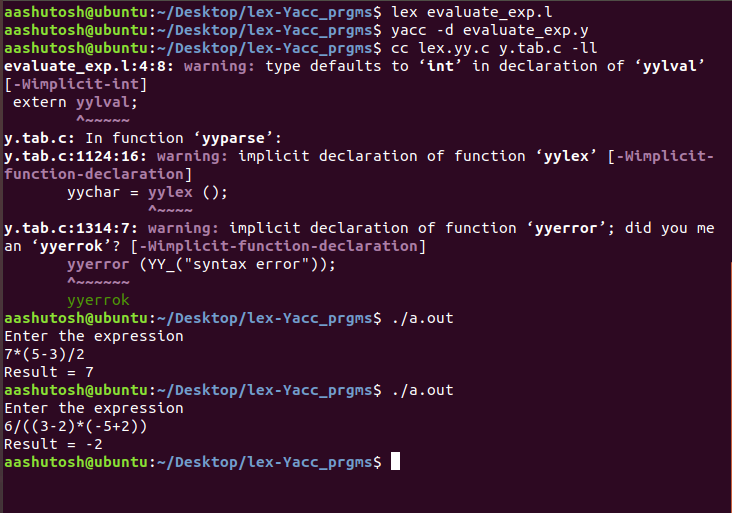# Yacc Program to evaluate a given arithmetic expression

Prerequisite – Introduction to YACC
Problem: Write a YACC program to evaluate a given arithmetic expression consisting of ‘+’, ‘-‘, ‘*’, ‘/’ including brackets.

Examples:

```Input: 7*(5-3)/2
Output: 7

Input: 6/((3-2)*(-5+2))
Output: -2 ```

Lexical Analyzer Source Code:

 `% ` ` ``{ ` `    ``/* Definition section*/` `    ``#include "y.tab.h" ` `    ``extern` `yylval; ` `    ``% ` ` ``} ` ` `  `% % ` `        ``[0 - 9] ` `    ``+ ` ` ``{ ` `    ``yylval = ``atoi``(yytext); ` `    ``return` `NUMBER; ` ` ``} ` ` `  `[a - zA - Z] + { ``return` `ID; } ` `[\t] + ; ` ` `  `\n { ``return` `0; } ` `. { ``return` `yytext; } ` ` `  `% %  `

Parser Source Code:

 `% { ` ` `  `/* Definition section */` `#include ` `     ``% ` ` `  `  ``} ` ` `  `% token NUMBER ID ` `// setting the precedence  ` `// and associativity of operators  ` `% left ``'+'` `'-'` `% left ``'*'` `'/'` ` `  `/* Rule Section */` `% ` `% E : T ` `  ``{ ` ` `  `  ``printf``(``"Result = %d\n"``, \$\$); ` `  ``return` `0; ` ` `  `  ``} ` ` `  `T : T ``'+'` `T { \$\$ = \$1 + \$3; } ` `| T ``'-'` `T { \$\$ = \$1 - \$3; } ` `| T ``'*'` `T { \$\$ = \$1 * \$3; } ` `| T ``'/'` `T { \$\$ = \$1 / \$3; } ` `| ``'-'` `NUMBER { \$\$ = -\$2; } ` `| ``'-'` `ID { \$\$ = -\$2; } ` `| ``'('` `T ``')'` `{ \$\$ = \$2; } ` `| NUMBER { \$\$ = \$1; } ` `| ID { \$\$ = \$1; }; ` `% % ` ` `  `    ``int` `main() ` ` ``{ ` `    ``printf``(``"Enter the expression\n"``); ` `    ``yyparse(); ` ` ``} ` ` `  `/* For printing error messages */` `int` `yyerror(``char``* s) ` ` ``{ ` `    ``printf``(``"\nExpression is invalid\n"``); ` ` ``} `

Output:Notes:
Yacc programs are generally written in 2 files one for lex with .l extension(for tokenization and send the tokens to yacc) and another for yacc with .y extension (for grammar evaluation and result evaluation).

Steps for execution of Yacc program:

```lex sample_lex_program.l
yacc -d sample_yacc_program.y
cc lex.yy.c y.tab.c -ll
./a.out
```

GeeksforGeeks has prepared a complete interview preparation course with premium videos, theory, practice problems, TA support and many more features. Please refer Placement 100 for details

My Personal Notes arrow_drop_upCheck out this Author's contributed articles.

If you like GeeksforGeeks and would like to contribute, you can also write an article using contribute.geeksforgeeks.org or mail your article to contribute@geeksforgeeks.org. See your article appearing on the GeeksforGeeks main page and help other Geeks.

Please Improve this article if you find anything incorrect by clicking on the "Improve Article" button below.

Article Tags :

Be the First to upvote.

Please write to us at contribute@geeksforgeeks.org to report any issue with the above content.